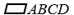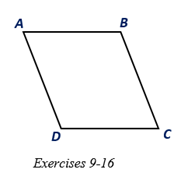Chapter 4.1, Problem 16E### Elementary Geometry for College St...

6th Edition
Daniel C. Alexander + 1 other
ISBN: 9781285195698

#### Solutions

Chapter
Section### Elementary Geometry for College St...

6th Edition
Daniel C. Alexander + 1 other
ISBN: 9781285195698
Textbook Problem
1 views

# Suppose that diagonals A C -   a n d   B D - ofare drawn and that A C > B D . Which angle ( ∠ A or ∠ B ) would have the greater measure?To determine

To describe:

The larger angle between A and B if AC>BD in.

Explanation

Given:

Diagonals AC- and BD- of are drawn and that AC>BD.

Corollary:

1. The opposite angles of a parallelogram are congruent.

2. Two consecutive angles of a parallelogram are supplementary.

3. The angle opposite to the larger side is larger than the angle opposite to the smaller side.

Calculation:

In the given parallelogram ABCD, given that AC>BD.

Join the diagonals AC- or BD- in

### Still sussing out bartleby?

Check out a sample textbook solution.

See a sample solution

#### The Solution to Your Study Problems

Bartleby provides explanations to thousands of textbook problems written by our experts, many with advanced degrees!

Get Started